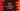# How to check if a swift dictionary is empty or notSwift dictionary provides isEmpty property to check if a dictionary is empty or not. This is a boolean property to indicate whether a dictionary is empty or not.

Other than that, we can also check the length of a dictionary to determine if it contains values. Dictionary property count returns the count of values. If it is zero, we can say that the dictionary is empty.

## Example with isEmpty :

Let’s consider the below example :

``````var first_dict = ["two": 2, "four": 4]

var second_dict: [String:Int] = [:]

if(first_dict.isEmpty){
print("first_dict is empty !")
}

if(second_dict.isEmpty){
print("second_dict is empty !")
}``````

In this example, we have two dictionaries : firstdict_ and seconddict_ where, seconddict_ is empty. We are checking the value of isEmpty for both dictionaries and printing one message if it is empty.

It prints the below output :

``second_dict is empty !``

i.e. isEmpty for seconddict_ is true.

## Example with count :

Alternatively, we can check the count of a dictionary and call it empty if the count is 0.

Let me change the above example with count :

``````var first_dict = ["two": 2, "four": 4]

var second_dict: [String:Int] = [:]

if(first_dict.count == 0){
print("first_dict is empty !")
}

if(second_dict.count == 0){
print("second_dict is empty !")
}``````

It prints the same output.